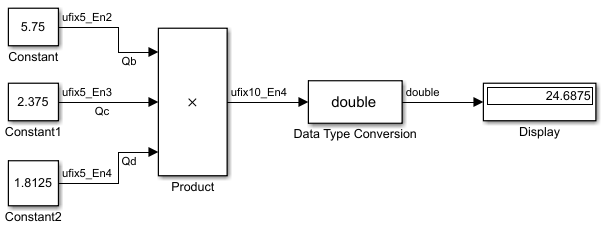## The Multiplication Process

The multiplication of an n-bit binary number with an m-bit binary number results in a product that is up to m + n bits in length for both signed and unsigned words.

Suppose you want to multiply three numbers. Each of these numbers is represented by a 5-bit word, and each has a different binary-point-only scaling. Additionally, the output is restricted to a 10-bit word with binary-point-only scaling of 2-4. The multiplication is shown in the following model for the input values 5.75, 2.375, and 1.8125.Applying the rules from the previous section, the multiplication follows these steps:

1. The first two numbers (5.75 and 2.375) are multiplied:

Note that the binary point of the product is given by the sum of the binary points of the multiplied numbers.

2. The result of step 1 is converted to the output data type:

`$\begin{array}{c}{Q}_{Temp}=convert\left({Q}_{RawProduct}\right)\\ =001101.1010=13.6250.\end{array}$`

Signal Conversions discusses conversions. Note that a loss in precision of one bit occurs, with the resulting value of QTemp determined by the rounding mode. For this example, round-to-floor is used. Furthermore, overflow did not occur but is possible for this operation.

3. The result of step 2 and the third number (1.8125) are multiplied:

Note that the binary point of the product is given by the sum of the binary points of the multiplied numbers.

4. The product is converted to the output data type:

`$\begin{array}{c}{Q}_{a}=convert\left({Q}_{RawProduct}\right)\\ =011000.1011=24.6875.\end{array}$`

Signal Conversions discusses conversions. Note that a loss in precision of 4 bits occurred, with the resulting value of QTemp determined by the rounding mode. For this example, round-to-floor is used. Furthermore, overflow did not occur but is possible for this operation.

Blocks that perform multiplication include the Product, Discrete FIR Filter, and Gain blocks.